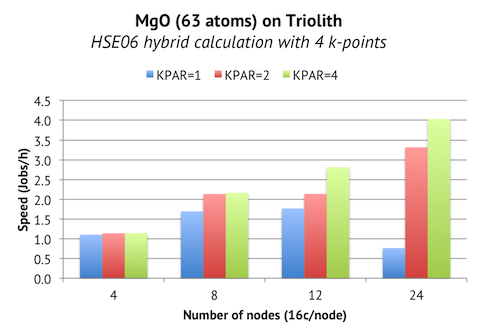# K-point Parallelization in VASP, Part 2

Previously, I tested the k-point parallelization scheme in VASP 5.3 for a small system with hundreds of k-points. The outcome was acceptable, but less than stellar. Paul Kent (who implemented the scheme in VASP) suggested that it would be more instructive to benchmark medium to large hybrid calculations with just a few k-points, since this was the original use case, and consequently where you would be able to see the most benefit. To investigate this, I ran a 63-atom MgO cell with HSE06 functional and 4 k-points over 4 to 24 nodes:A suitable number of bands here is 192, so the maximum number of nodes we could expect to use with standard parallelization is 12, due to the fact that 12 nodes x 16 cores/node = 192 cores. And we do see that KPAR=1 flattens out at 1.8 jobs/h on 12 nodes. But with k-point parallelization, the calculation can be split into “independent” groups, each running on 192 cores. This enables us, for example, to run the job on 24 nodes using KPAR>=2, which in this case translates into a doubling of speed (4.0 jobs/h), compared to the best case scenario without k-point parallelization.

So there is indeed a real benefit for hybrid calculations of cells that are small enough to need a few k-points. And remember that in order for the k-point parallelization to work correctly with hybrids, you should set:

``````NPAR = total number of cores / KPAR.
``````